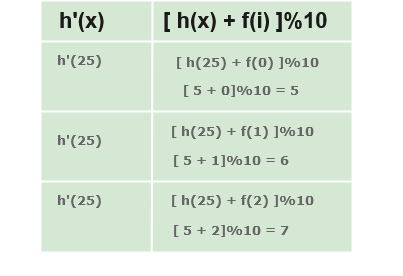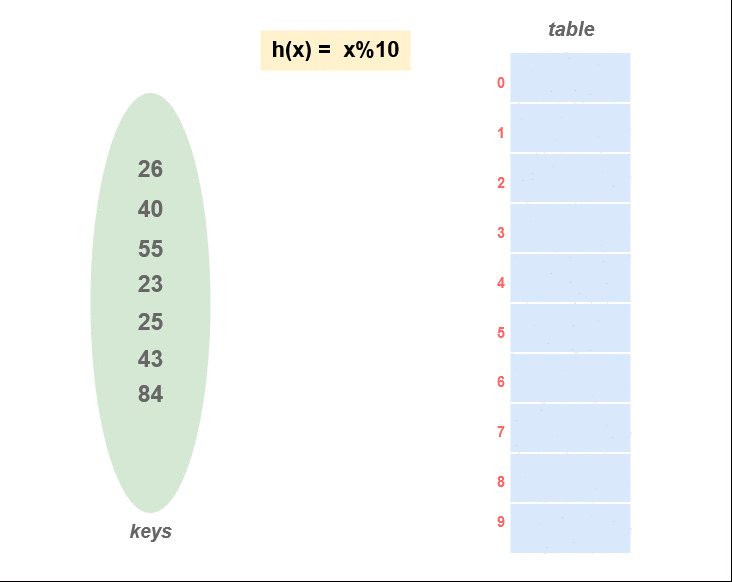# Open Addressing

Open addressing is a collision resolution technique in which a method known as probing is used to make efficient use of the hash table’s space.
All elements are stored in the hash table itself in open addressing.
Unlike chaining, multiple elements cannot be stored in the same slot in the hash table.

Probing: A collision is resolved by searching through alternate locations in the hash table until either the target key is located and the data is stored there itself. If some value is already present, then the information is stored at the next available space.This method is known as Probing.
Open addressing is also known as Closed hashing.

Note that the size of the hash table must be greater than or equal to the total number of keys.

Steps followed in Open addressing :

• Keys are generated using a hash function.
• Go to the required generated index to find if it is empty or not.
• If the index is empty, then store data at that index.
• If there is a value already present at that location, then store data at the next available space.

In this way, keys can be stored without any extra pointer or a linked list.
In open addressing technique, each slot is either filled with a single key or left empty or nil.

The methods used by open addressing are:

• Linear Probing
• Quadratic Probing
• Double Hashing

## Linear Probing

We search for the next empty location by looking into the next slot until we find an empty slot. This technique is called linear probing.

The general hash function used in linear probing is : `h'(x) = (h(x) + f(i))%size`
where, `i = 0, 1, 2, ….` and `h'(k)` is the new hash function.
The value of `i` is incremented linearly.

Hash Function :

``````int hash(int key)
{
return key%SIZE;
}``````

Probe Function :

``````int probe(int H[],int key)
{
int index=hash(key);
int i=0;
while(H[(index+i)%SIZE]!=0)
i++;
return (index+i)%SIZE;
}``````

## Insertion

• Get the hash code of the key passed
• Locate the index using that hash code as an index
• If location has no data present, insert data.
• Use linear probing if an element is found at the computed hash code.``````void Insert(int H[],int key)
{
int index=hash(key);
if(H[index]!=0)
index=probe(H,key);
H[index]=key;
}``````

## Searching

• Get the hash code of the key passed
• Locate the element using that hash code as index.
• Use linear probing to get the element ahead if the element is not found at the computed hash code.
``````int Search(int H[],int key)
{
int index=hash(key);
int i=0;
while(H[(index+i)%SIZE]!=key)
i++;
return (index+i)%SIZE;
}``````

One issue with linear probing is that a cluster of adjacent slots gets filled. While inserting a new element, this entire cluster has to be traversed which increases the time required to perform operations on the hash table.
Quadratic Probing and Double Hashing find methods to reduce the size of the clusters that are formed by linear probing.

## Quadratic Probing

In quadratic probing, the spacing between the slots is made to be more than one by using the a particular function.
The function used in quadratic probing is : `h(k, i) = (h′(k) + c1i + c2i2)%m`
where,

• `c1` and `c2` are positive auxiliary constants,
• `i = 0, 1, ….`

To make sure that the quadratic probes hit every single available spots, the table size must meet be:

• a prime number
• never more than half full (even by one element)
• ## Double hashing

Whenever a collision occurs, another slot is chosen in the table to put the value. But, in double hashing, instead of choosing next frees lot, a second hash function is used to determine the location of the next slot.

## Analysis of Open Addressing

Like Chaining, the performance of hashing is evaluated using the loading function :

If n = Number of keys to be inserted in the hash table
Loading factor λ = `n/size ( < 1 )`

Expected time to search/insert/delete `< 1/(1 - λ )`

So Search, Insert and Delete take `(1/(1 - λ ))` time

Scroll to Top

### Full Stack Integrated Bootcamp Free Trial

• Please enter a number from 7000000000 to 9999999999.